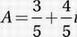# SAT Math Multiple Choice Question 613: Answer and Explanation

### Test Information

Question: 613

13. If the complex number A satisfies the equation A(2 - i) = 2 + i, where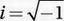, what is the value of A?

• A. 5 - i
• B. 5 + i
• C.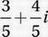• D.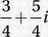Explanation:

C

Special Topics (complex numbers) HARD

A(2 - i) = 2 + i

Divide by (2 - i):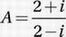Multiply numerator and denominator by the conjugate (2 + i):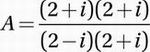FOIL: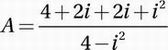Combine terms: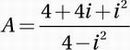Substitute i2 = - 1: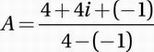Simplify: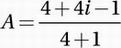Combine terms: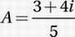Distribute to express in standard a + bi form: